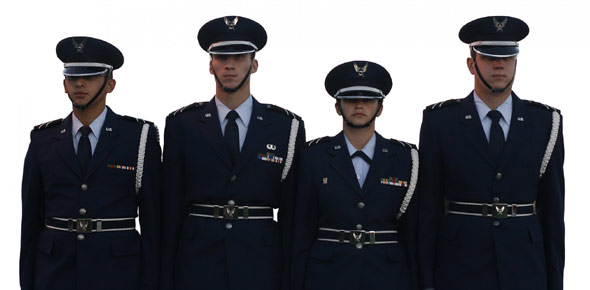# Air Force Quiz

12 Questions | Total Attempts: 75.

• 1.
What is the six-letter Phrase that should be remembered by bootcamp?
• A.

Ma'am airman o'connell here as ordered

• B.

Ma'am trainee o'connell her as ordered

• C.

Ma'am trainee o'connell reports as ordered

• 2.
How many bars does an airman (AMN) E-2 have?
• A.

One

• B.

Four

• C.

Two

• 3.
How many bars does an airman first class (A1C) E-3 have?
• A.

Three

• B.

One

• C.

Two

• 4.
How many bars does an Staff Sergeant (SSGT) E-5 have?
• A.

Four

• B.

Six

• C.

Two

• 5.
How many bars does an technical Sergeant (TSGT) E-6 have?
• A.

Five

• B.

Sever

• C.

Four

• 6.
How many bars does an Master sergeant (MAGT) E-7 have?
• A.

Five

• B.

Six

• C.

Seven

• 7.
How many bars does an first sergeant (1sgt) E-7 have?
• A.

Six with a sideways dimoand

• B.

Six with a up and down dimoand

• C.

Six with no dimoand

• 8.
How many bars does an senior Master SGT (SMSGT) E-8 have?
• A.

Six with dimoand

• B.

Eight with sideways dimoand

• C.

Seven

• 9.
How many bars does an chief master sergeant (CMSGT) E-9 have?
• A.

Eight with a dimoand

• B.

Eight

• C.

Seven

• 10.
How many bars does an First Sergeant (1SGT) E-9 have?
• A.

Eight with an sideways dimoand

• B.

Eight

• C.

Nine

• 11.
How many bars does an Comman Chief Master SGT (CCM) E-9 have?
• A.

Eight with an dimoand

• B.

Eight with a star

• C.

Eight

• 12.
How many bars does an Chief MSGT Of The Air Force (1SGT) E-9 have?
• A.

Eight with dimoand

• B.

Eight

• C.

Eight with AF star at bottom

Related Topics